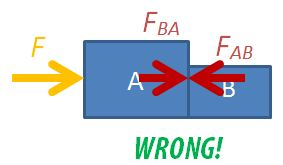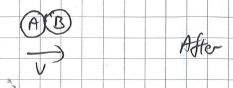## Can a Liquid Freeze and Boil Simultaneously?

Witness the freezing and boiling of a liquid take place at the same time. A thorough explanation for this observation is found here.

## Which Equations Apply Only to Ideal Gases?

Students are sometimes unclear about which of the equations taught in the topic of Thermal Physics apply to ideal gases and which apply to all systems (whether ideal or real gas, even liquids and solid). The following table should help to clarify:

 Applies to Ideal Gas only Applies to all systems Gas Laws $pV=nRT$ $pV\approx nRT$ only for gases at low pressure and high temperatures Average Kinetic Energy $=\dfrac{3}{2}kT$ $\propto T$ Internal Energy $U$ = sum of KE of molecules$U=\dfrac{3}{2}NkT=\dfrac{3}{2}nRT=\dfrac{3}{2}pV$ $U$ = sum of KE and PE of molecules First Law of Thermodynamics applies to all systems $\Delta U=Q+W$

## 3 Common Dynamics Mistakes

After going through the topic of Dynamics in the A-level syllabus recently, it strikes me that there are several common mistakes or misconceptions that students whom I have taught over the years have always struggled with. I shall lay them out here in the hope that students who read this do not repeat the same mistakes.

### 1. Mixing up free-body diagrams involving multiple bodies in motion

Students are often confused about the forces in drawing free-body diagrams, especially so when they have to consider the different parts of multiple bodies in motion. A common mistake that students make is to draw all the forces, including the internal forces within a system, on a single diagram.To solve a two-body dynamics problems, students need to learn how to draw the 3 possible free-body diagrams. A detailed set of instructions is found in an earlier post.

Students who do not have a strong foundation in Math may not appreciate the fact that the addition and subtracting of vectors is not done by simply adding or subtracting the magnitudes. Sometimes, students are asking to find the force acting on an object when given the initial and final momenta.  The correct way to find the vector of the change in momentum is to place the tails of the initial and final momenta together, and to join the arrowhead of the initial momentum with that of the final momentum with a third vector in that direction.

They are often confused between finding the change in momentum and the act of adding two vectors together. When that happens, what teachers see is the following triangle instead.

While the magnitude of the vector found in both diagrams are the same, students will get the wrong answer if asked for the direction of the change in momentum. This is actually an important notion because the direction of change in momentum is really the direction of the net force applied on the body.

### 3. Using wrong signs for momentum for head-on collisions

In A-level physics, in the study of collision problems, we will deal only with head-on collisions where two objects collide along the same line. Even then, students can get confused with the sign convention associated with the direction of motion of the objects.

###### Applying Conservation of Momentum

For a two body head-on collision problem, always start by writing down the following equation for the conservation of momentum:

$m_Au_A +m_Bu_B = m_Av_A + m_Bv_B$

where $m_X$ is the mass of X, $u_X$ is the initial velocity of X,  $v_X$ is the final velocity of X, where X is either A or B.Follow this up by deciding on which direction you want to define as positive. For example, if you decide that the rightward direction is positive, the value of initial velocity for mass A is positive and that for mass B is negative.

What students sometimes do is to include the negative signs into the equation before substitution, e.g. $m_Au_A +m_B(-u_B) = m_Av_A + m_Bv_B$. This causes confusion as they do not know where the directions of the final velocities are and hence, do not know which vectors to assign as negative.

What we should do instead is to determine the final direction of the velocities after we have found the values. Before we are able to do so, we shall assume that the final velocities are to the right. If the final value of velocity of a mass is negative, it means that its final velocity is to the left.

###### Substitute the values into equation.

Assuming that the initial speeds are both 4.0 m s-1;, the mass of A is 3.0 kg, mass of B is 5.0 kg, and that the collision is perfectly inelastic, we have:$3.0 \times 4.0+5.0 \times(-4.0)=(3.0+5.0) \times v$
$-8.0=8.0v$
$v=-1.0 \text{ m s}^{-1}$

This way, since the final velocity of the objects has a negative value, we can tell that the direction of the final velocity is to the left.

## Free-Body Diagrams in Two-Body Motion

Students are often confused about the forces in drawing free-body diagrams, especially so when they have to consider the different parts of multiple bodies in motion.

##### Two-Body Motion

Let's consider the case of a two-body problem, where, a force F is applied to push two boxes horizontally. If we were to consider the free-body diagram of the two boxes as a single system, we only need to draw it like this.

For the sake of problem solving, there is no need to draw the normal forces or weights since they cancel each other out, so the diagram can look neater. Applying Newton's 2nd law of motion, $F=(m_A+m_B) \times a$, where $m_A$ is the mass of box A, $m_B$ is the mass of box B, F is the force applied on the system and a is the acceleration of both boxes.

You may also consider box A on its own.

The equation is $F-F_{AB}=m_A \times a$, where $F_{AB}$ is the force exerted on box A by box B.

The third option is to consider box B on its own.

The equation is $F_{BA}=m_B \times a$, where $F_{BA}$ is the force exerted on box B by box A. Applying Newton's 3rd law, $F_{BA}=F_{AB}$ in magnitude.

##### Never Draw Everything Together

NEVER draw the free-body diagram with all the forces and moving objects in the same diagram, like this:You will not be able to decide which forces acting on which body and much less be able to form a sensible equation of motion.

##### Multiple-Body Problems

For the two-body problem above, we can consider 3 different free-body diagrams. For a three bodies in motion together, we can consider up to 6 different free-body diagrams: the 3 objects independently, 2 objects at a go, and all 3 together.

[WpProQuiz 2]

Here are some interesting lecture demonstrations on adiabatic thermodynamic processes you can carry out. In an adiabatic process, there is no heat transfer between the system and other systems (including its environment.) According to the First Law of Thermodynamics ($\Delta U=Q+W$), where Q = 0, a compression of a gas which is associated with work being done on the gas will cause the internal energy and hence, the temperature of the gas to rise. On the other hand, when an expansion of a gas takes place, the gas will cool down.

##### 1. Adiabatic compression using a fire syringe

(available from Funlearners for \$30 in Singapore)